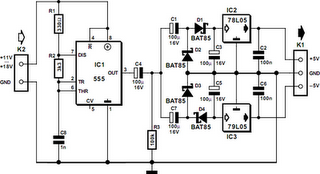# Learning Electronics

Learn to build electronic circuits

# 555 DC/DC Converter

It is all too often necessary to augment the power supply of an existing electronic circuit because exactly the voltage that you need is missing. The circuit presented here may provide a solution in a number of cases, since it can be used to convert a single-ended supply voltage into a balanced set of supply voltages. That’s not so remarkable by itself, but the special feature of this circuit is that this is accomplished without using difficult to obtain, exotic ICs. All of the components used in the circuit are ones that every electronics hobbyist is likely to have in a drawer somewhere.

The heart of the circuit is formed by an ‘old reliable’ 555 timer, which is wired here as a free-running oscillator with a frequency of approximately 160 kHz. The oscillator is followed by two voltage-doubling rectifiers, consisting of C1, D1, D2, C3 and C7, D3, D4, C5. They are followed in turn by two voltage regulators to stabilise the positive and negative voltages generated in this manner. The duty cycle of the 555 is set to approximately 50 percent using R1 and R2. The square-wave signal at the output of the timer IC has a DC offset, which is eliminated by C4 and R3.555 DC/DC Converter Circuit Diagram

The amplitude of the output signal from the 555 is approximately equal to the supply voltage less 1.5 V, so with a 12-V input voltage, there will be a square-wave signal on pin 3 with an amplitude of approximately 10.5 Vpp. With respect to ground (across R3), this is this +5 V / –5 V. Although this yields a symmetric voltage, its positive and negative amplitudes are somewhat too small and it is not stabilised. In order to split the square-wave signal into sufficiently large positive and negative amplitudes, C1/D2 are added for the positive voltage, causing the positive half to be doubled in amplitude.

For the negative half, the same effect is achieved using C7/D3. Following this, the two signals are smoothed by D1/C3 and D4/C5, respectively. Both voltages are now high enough to be input to normal 5-V voltage regulators, yielding symmetric +5V and –5V supply voltages at the output. The input voltage does not have to be regulated, although it must lie between +11 V and +18 V. The maximum output current is ±50 mA with an input voltage of 12 V. This circuit is an excellent choice for generating auxiliary voltages, such as supply voltages for low-power opamps. Naturally, the fact that the converter can be powered from the in-vehicle voltage of a car is a rather attractive feature.
Author: L. de Hoo - Copyright: Elektor Electronics